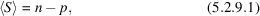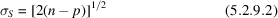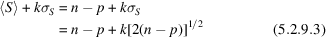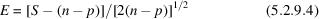International
Tables for
Crystallography
Volume C
Mathematical, physical and chemical tables
Edited by E. Prince

International Tables for Crystallography (2006). Vol. C, ch. 5.2, p. 498

## Section 5.2.9. Testing for remanent systematic error

W. Parrish,a A. J. C. Wilsonb and J. I. Langfordc

aIBM Almaden Research Center, San Jose, CA, USA,bSt John's College, Cambridge CB2 1TP, England, and cSchool of Physics & Astronomy, University of Birmingham, Birmingham B15 2TT, England

### 5.2.9. Testing for remanent systematic error

| top | pdf |

Since about 1930, it has been claimed that the lattice parameters of cubic substances could be measured within one part in 50 000. Precision (that is, reproducibility of measurements by one technique within one laboratory) of this order is achieved, but accuracy (agreement between determinations by different techniques or by the same technique in different laboratories) is lower. The IUCr lattice-parameter project (Parrish, 1960) showed a standard deviation of 1 in 30 000 in inter-laboratory comparisons, with some outlying values differing from the mean by one or two parts in 10 000. At that time, therefore, precision was considerably better than accuracy (absence of significant remanent systematic error). Testing for remanent systematic error is thus valuable as an occasional test of methodology, though not undertaken as routine. The principle is outlined here, and more details are given in Chapters 8.4and 8.5.

When refinement of parameters is performed by least squares, weighted in accordance with the reciprocal of the estimated variance, the expected value of the weighted sum of squares iswhere n is the number of terms summed and p is the number of parameters determined. The standard deviation of the sum S is expected to beapproximately (Wilson, 1980), so that if the actual value of S exceeds(where k = 2 or 3), one can reasonably conclude that there are defects in the model (remanent systematic errors). If S is less than this value, one can reasonably conclude that any defects in the model (systematic errors) are at worst of the same order of magnitude as the statistical fluctuations; the sensitivity of the test increases rather slowly with np. The method was advocated by Beu and his collaborators (Beu, Musil & Whitney, 1962, 1963; Beu, 1964; Beu & Whitney, 1967; Langford, Pike & Beu, 1964; see also Mitra, Ahmed & Das Gupta, 1985) because tests of the hypothesis `no remaining systematic error' based on likelihood were available; they assumed a normal distribution of errors, possibly without realizing, and certainly without emphasizing, that the method was then equivalent to least squares. Their application of the method to testing for remanent systematic error in lattice-parameter determination was successful: the aberrations of the counter diffractometer were found to be adequately accounted for: additional aberrations were found for the Bond method (see Chapter 5.3); Boom (1966) used it in testing the accuracy of the Debye–Scherrer method.

In statistical literature, the weighted sum of squares S is often called the scaled deviance, andis called the excess. The test for the absence of significant systematic error is then that the excess should be less than three.

### References

Beu, K. E. (1964). The evaluation of centroid lattice parameter data for tungsten by the likelihood ratio method. Acta Cryst. 17, 1149–1164.
Beu, K. E., Musil, F. J. & Whitney, D. R. (1962). Precise and accurate lattice parameters by film powder methods. I. The likelihood ratio method. Acta Cryst. 15, 1292–1301.
Beu, K. E., Musil, F. J. & Whitney, D. R. (1963). The likelihood ratio method for the precise and accurate determination of lattice parameters for tetragonal and hexagonal crystals. Acta Cryst. 16, 1241–1242.
Beu, K. E. & Whitney, D. R. (1967). Further developments in a likelihood ratio method for the precise and accurate determination of lattice parameters. Acta Cryst. 22, 932–933.
Boom, G. (1966). Accurate lattice parameters and the LPC method. Groningen: van Denderen.
Langford, J. I., Pike, E. R. & Beu, K. E. (1964). Precise and accurate lattice parameters by film powder methods. IV. Theoretical calculation of axial (vertical) divergence profiles, centroid shifts, and variances for cylindrical powder diffraction cameras. Acta Cryst. 17, 645–651.
Mitra, G. B., Ahmed, A. & Das Gupta, P. (1985). Precise and accurate estimation of crystallographic parameters by maximum-likelihood and min–max methods. Structure and statistics in crystallography, edited by A. J. C. Wilson, pp. 151–181. Guilderland, NY: Adenine Press.
Parrish, W. (1960). Results of the IUCr precision lattice-parameter project. Acta Cryst. 13, 838–850.
Wilson, A. J. C. (1980). Accuracy in methods of lattice-parameter measurement. Natl Bur. Stand. (US) Spec. Publ. No. 567, pp. 325–351.